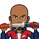# MACD + SMA 200 Strategy (by ChartArt)

40991 뷰
40991
Here is a combination of the classic MACD (moving average convergence divergence indicator) with the classic slow moving average SMA with period 200 together as a strategy.

This strategy goes long if the MACD histogram and the MACD momentum are both above zero and the fast MACD moving average is above the slow MACD moving average. As additional long filter the recent price has to be above the SMA 200. If the inverse logic is true, the strategy goes short. For the worst case there is a max intraday equity loss of 50% filter.

Save another \$999 bucks with my free strategy.

This strategy works in the backtest on the daily chart of Bitcoin , as well as on the S&P 500 and the Dow Jones Industrial Average daily charts . Current performance as of November 30, 2015 on the SPX500 CFD daily is percent profitable: 68% since the year 1970 with a profit factor of 6.4. Current performance as of November 30, 2015 on the DOWI index daily is percent profitable: 51% since the year 1915 with a profit factor of 10.8.

All trading involves high risk; past performance is not necessarily indicative of future results. Hypothetical or simulated performance results have certain inherent limitations. Unlike an actual performance record, simulated results do not represent actual trading. Also, since the trades have not actually been executed, the results may have under- or over-compensated for the impact , if any, of certain market factors, such as lack of liquidity. Simulated trading programs in general are also subject to the fact that they are designed with the benefit of hindsight. No representation is being made that any account will or is likely to achieve profits or losses similar to those shown.
```//@version=2
strategy("MACD + SMA 200 Strategy (by ChartArt)", shorttitle="CA_-_MACD_SMA_strategy", overlay=true)

// ChartArt's MACD + SMA 200 Strategy
//
// Version 1.0
// Idea by ChartArt on November 30, 2015.
//
// Here is a combination of the MACD with the
// slow moving average SMA 200 as a strategy.
//
// This strategy goes long if the MACD histogram
// and the MACD momentum are both above zero and
// the fast MACD moving average is above the
// slow MACD moving average. As additional long filter
// the recent price has to be above the SMA 200.
// If the inverse logic is true, the strategy
// goes short. For the worst case there is a
// max intraday equity loss of 50% filter.

// Input
source = input(close)
fastLength = input(12, minval=1, title="MACD fast moving average")
slowLength=input(26,minval=1, title="MACD slow moving average")
signalLength=input(9,minval=1, title="MACD signal line moving average")
veryslowLength=input(200,minval=1, title="Very slow moving average")
switch1=input(true, title="Enable Bar Color?")
switch2=input(true, title="Enable Moving Averages?")
switch3=input(true, title="Enable Background Color?")

// Calculation
fastMA = sma(source, fastLength)
slowMA = sma(source, slowLength)
veryslowMA = sma(source, veryslowLength)
macd = fastMA - slowMA
signal = sma(macd, signalLength)
hist = macd - signal

// Colors
MAtrendcolor = change(veryslowMA) > 0 ? green : red
trendcolor = fastMA > slowMA and change(veryslowMA) > 0 and close > slowMA ? green : fastMA < slowMA and change(veryslowMA) < 0 and close < slowMA ? red : blue
bartrendcolor = close > fastMA and close > slowMA and close > veryslowMA and change(slowMA) > 0 ? green : close < fastMA and close < slowMA and close < veryslowMA and change(slowMA) < 0 ? red : blue
backgroundcolor = slowMA > veryslowMA and crossover(hist, 0) and macd > 0 and fastMA > slowMA and close[slowLength] > veryslowMA ? green : slowMA < veryslowMA and crossunder(hist, 0) and macd < 0 and fastMA < slowMA and close[slowLength] < veryslowMA ? red : na
bgcolor(switch3?backgroundcolor:na,transp=80)
barcolor(switch1?bartrendcolor:na)

// Output
F=plot(switch2?fastMA:na,color=trendcolor)
S=plot(switch2?slowMA:na,color=trendcolor,linewidth=2)
V=plot(switch2?veryslowMA:na,color=MAtrendcolor,linewidth=4)
fill(F,V,color=gray)

// Strategy
buyprice = low
sellprice = high
cancelLong = slowMA < veryslowMA
cancelShort = slowMA > veryslowMA

if (cancelLong)
strategy.cancel("MACDLE")

if crossover(hist, 0) and macd > 0 and fastMA > slowMA and close[slowLength] > veryslowMA
strategy.entry("MACDLE", strategy.long, stop=buyprice, comment="Bullish")

if (cancelShort)
strategy.cancel("MACDSE")

if crossunder(hist, 0) and macd < 0 and fastMA < slowMA and close[slowLength] < veryslowMA
strategy.entry("MACDSE", strategy.short, stop=sellprice, comment="Bearish")

maxIdLossPcnt = input(50, "Max Intraday Loss(%)", type=float)
strategy.risk.max_intraday_loss(maxIdLossPcnt, strategy.percent_of_equity)

//plot(strategy.equity, title="equity", color=red, linewidth=2, style=areabr)```Amazing indicator - I can't live without this on my chart

Is there a way to edit the "Strategy Tester" out of the code?
I'd like to use it solely as a chart indicator

Cheers!
응답the bartrend color setup is confusing to me... what am i missing? I can't figure out what the color changes mean in relation to the bars, signal, trend, etc... could someone please explain to me how and why the bars go from blue to green and blue to red, etc, and what it means when it happens? thanks
응답